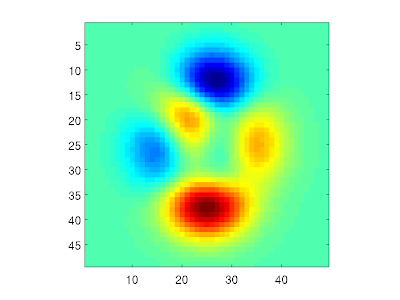## Monday, 10 May 2010

### The curse of Matlab NaNs

NaN is a special kind of Matlab value, representing Not-A-Number. This is often returned from a function or operation where the output is not well defined. For example, if you try to interpolate a value outside of the range of x values, the interp1 function returns NaN for this value. The following sample code illustrates this:

x = [1 2];
y = [1 4];
xi = 5;
y = interp1(x,y,xi)

returns y = NaN.

You may not always notice that a variable contains NaN values. For example, let's plot the "peaks" matrix from Matlab as an image:

X = peaks;
imagesc(X)Now, if we set the middle element equal to NaN, this will show up as a blue spot in the image. This is because Matlab plots NaN elements with the "lowest" colormap color:

X(25,25) = NaN;
imagesc(X)
colorbarNote here that there is no way of distinguishing a NaN from a valid data value in such an image. However, we can check how many NaN elements there is in a variable:

numberOfNanElements = nnz(isnan(X))

which returns 1. Now, NaN has the unfortunate property that is "taints" all other elements that are affected by it. For example, if we take a 2D Fourier transform of X, all the elements returned by the function are NaN. So,

nnz(isnan(fft2(X)))

returns 2410! One single NaN pixel in the original image makes the entire transform unusable. Although I can understand the logic behind this behavior, it has been (and still is) a frequent source of very frustrating bugs in my work. When debugging, I often check 2D datasets by plotting them as images -- but as we have seen, a few NaN values can easily be present in an image without standing out from the valid data points. So, thinking that the dataset is valid, I continue stepping through the code, only to find that the complete dataset suddenly turns into NaNs. In this situation it is easy to conclude that there is something wrong in the last function used, when the fault really lies in the input data.

I've spent too many hours agonizing over such errors, so here's a tip for everyone that think that NaNs are messing up their code: For all variables that may potentially contain NaNs, set all NaN elements to zero, like this:

X(isnan(X)) = 0;

A zero value may not necessarily be "correct" as such, but it doesn't have the potential of NaNs to destroy the complete dataset. Now, if I could only remember this the next time I encounter "the curse of the NaNs"... ;-)